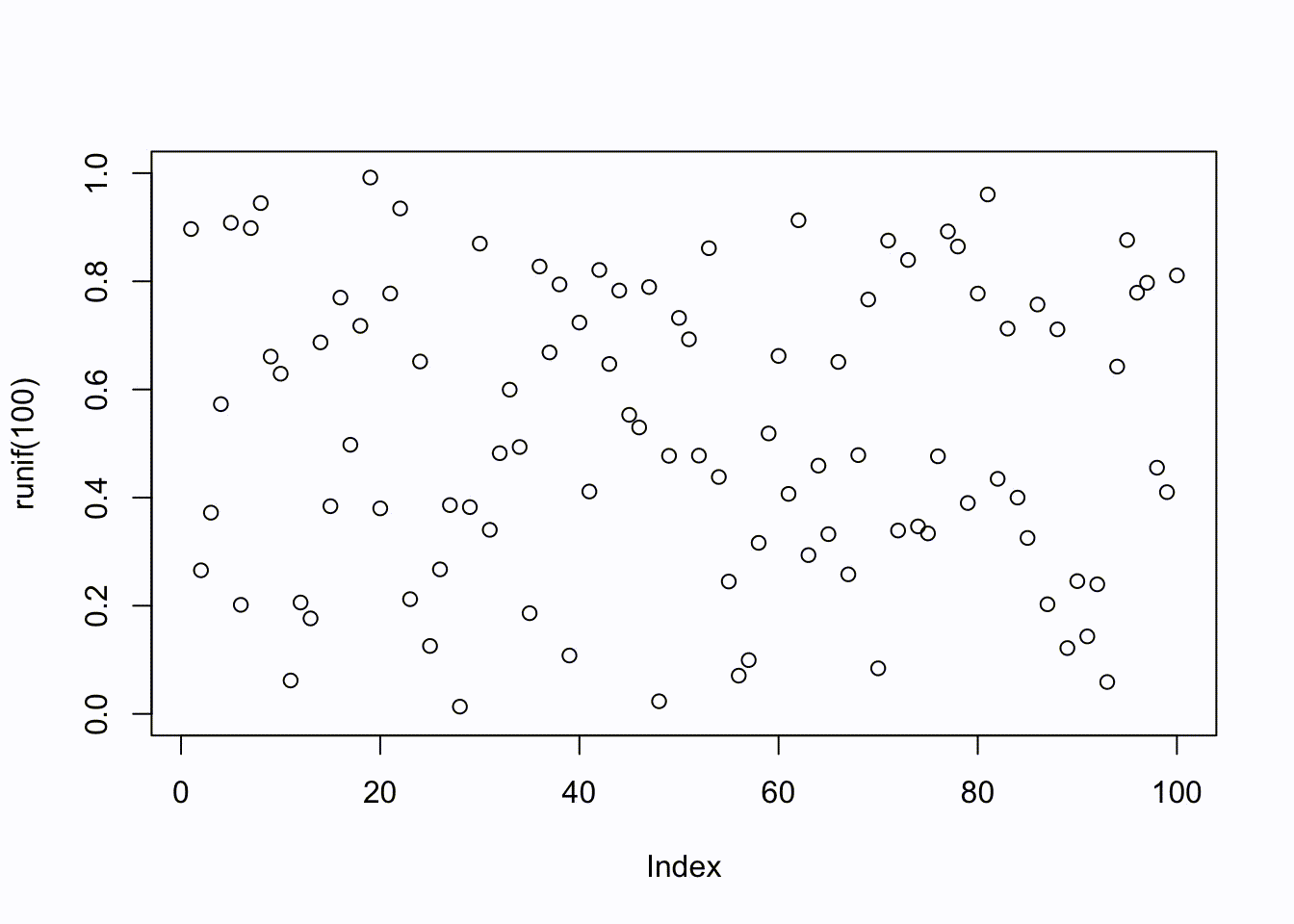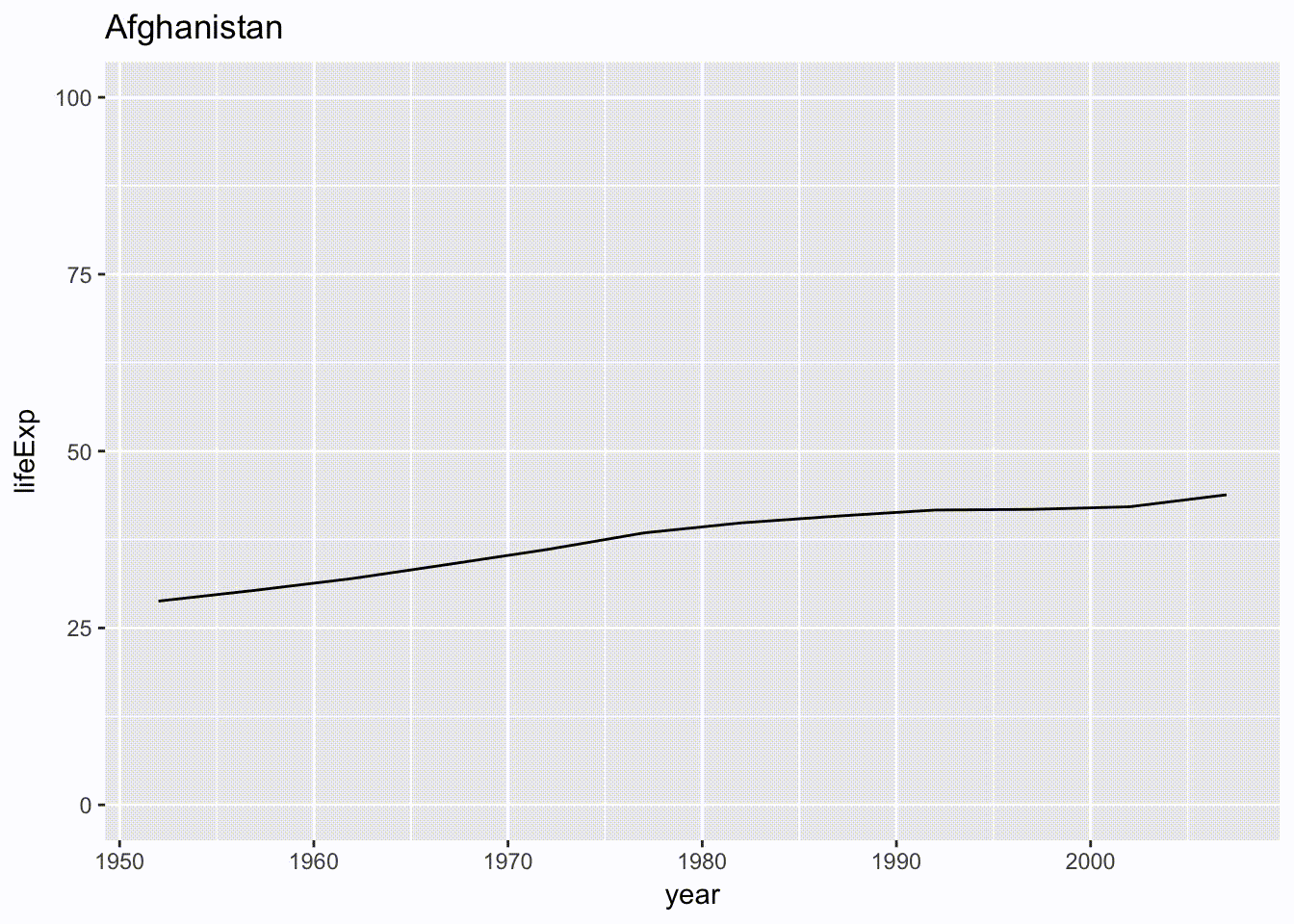This post will show you how to include GIFs in a blogdown post from a website, and how to make new GIFs from R plots (base R and ggplot2) using knitr chunk options.

# Embed plot GIFs using R

To create a GIF animation of a plot made in R, you need to install FFmpeg on your computer. If you are on a macOS, you can install FFmpeg through Homebrew using the formula `brew install ffmpeg`.

You can make a GIF animation using knitr starting in version 1.15. A GIF animation of the plots in a code chunk will be generated for HTML output when you use the chunk options `fig.show = 'animate'`, `ffmpeg.format = 'gif'`, and `dev = 'jpeg'`.

Here is an example knitr chunk with options:

`````````{r chunk-label, fig.show='animate', ffmpeg.format='gif', dev='jpeg'}
for (i in 1:10) plot(runif(100), ylim = c(0, 1)) # for example
`````````

Which would produce the GIF below…Let’s break those chunk options down:

• `fig.show = 'animate'`: how to show/arrange the plots; `animate` wraps all plots into an animation if there are mutiple plots in a chunk

• `ffmpeg.format = 'gif'`: the filename of the extension video you want to create. This `.gif` file is also saved in your `/static/post/` directory.

• `dev = 'jpeg'`: the function name which will be used as a graphical device to record the individual plots that make up the above. These `.jpeg` files are each saved in your `/static/post/` directory.

You can also create GIFs using the purrr and ggplot2 packages.

``````library(purrr)
library(ggplot2)
library(repurrrsive) # for the example data below``````

Below is some sample code from an awesome purrr tutorial by Charlotte Wickham. From her `map2()` example code:

``````gap_split_small <- gap_split[1:10]
countries <- names(gap_split_small)
# For all countries
plots <- map2(gap_split_small, countries,
~ ggplot(.x, aes(year, lifeExp)) +
geom_line() +
labs(title = .y) +
coord_cartesian(ylim = c(0, 100))) ``````

To show these plots as an animation, we would use this code chunk:

`````````{r gapgif, fig.show='animate', ffmpeg.format='gif', dev='jpeg'}
# Display all plots
walk(plots, print)
`````````

Which produces the GIF below…